# Clustergram¶

## Visualization and diagnostics for cluster analysis¶

Clustergram is a diagram proposed by Matthias Schonlau in his paper The clustergram: A graph for visualizing hierarchical and nonhierarchical cluster analyses.

In hierarchical cluster analysis, dendrograms are used to visualize how clusters are formed. I propose an alternative graph called a “clustergram” to examine how cluster members are assigned to clusters as the number of clusters increases. This graph is useful in exploratory analysis for nonhierarchical clustering algorithms such as k-means and for hierarchical cluster algorithms when the number of observations is large enough to make dendrograms impractical.

The clustergram was later implemented in R by Tal Galili, who also gives a thorough explanation of the concept.

This is a Python translation of Tal’s script written for `scikit-learn` and RAPIDS `cuML` implementations of K-Means, Mini Batch K-Means and Gaussian Mixture Model (scikit-learn only) clustering.

## Getting started¶

You can install clustergram from `conda` or `pip`:

```conda install clustergram -c conda-forge
```
```pip install clustergram
```

In any case, you still need to install your selected backend (`scikit-learn` or `cuML`).

The example of clustergram on Palmer penguins dataset:

```import seaborn
```

First we have to select numerical data and scale them.

```from sklearn.preprocessing import scale
data = scale(df.drop(columns=['species', 'island', 'sex']).dropna())
```

And then we can simply pass the data to `clustergram`.

```from clustergram import Clustergram

cgram = Clustergram(range(1, 8))
cgram.fit(data)
cgram.plot()
```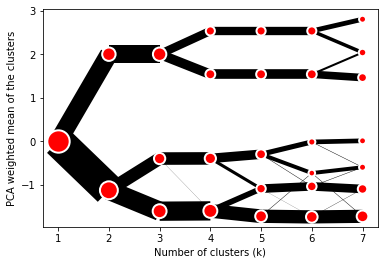## Styling¶

`Clustergram.plot()` returns matplotlib axis and can be fully customised as any other matplotlib plot.

```seaborn.set(style='whitegrid')

cgram.plot(
ax=ax,
size=0.5,
linewidth=0.5,
cluster_style={"color": "lightblue", "edgecolor": "black"},
line_style={"color": "red", "linestyle": "-."},
figsize=(12, 8)
)
```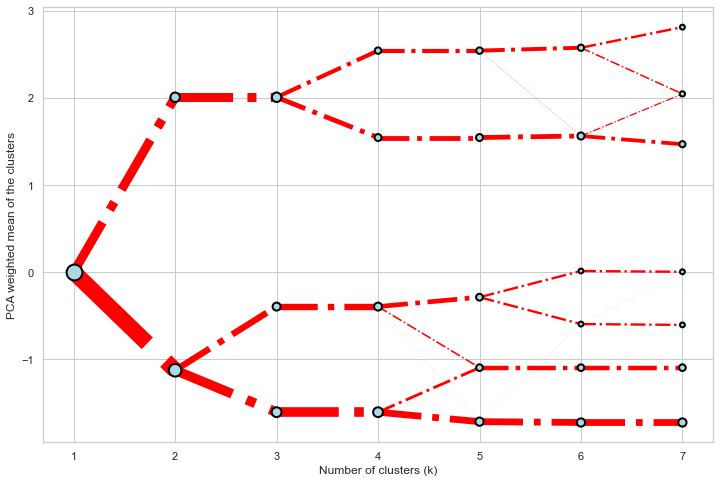## Mean options¶

On the `y` axis, a clustergram can use mean values as in the original paper by Matthias Schonlau or PCA weighted mean values as in the implementation by Tal Galili.

```cgram = Clustergram(range(1, 8))
cgram.fit(data)
cgram.plot(figsize=(12, 8), pca_weighted=True)
```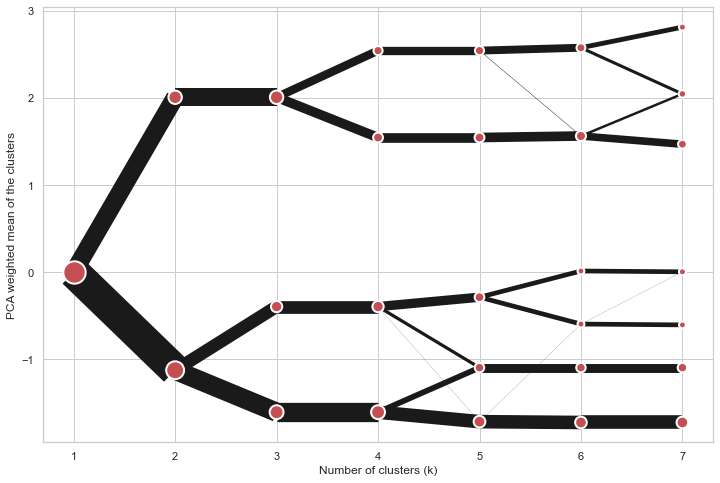```cgram = Clustergram(range(1, 8))
cgram.fit(data)
cgram.plot(figsize=(12, 8), pca_weighted=False)
```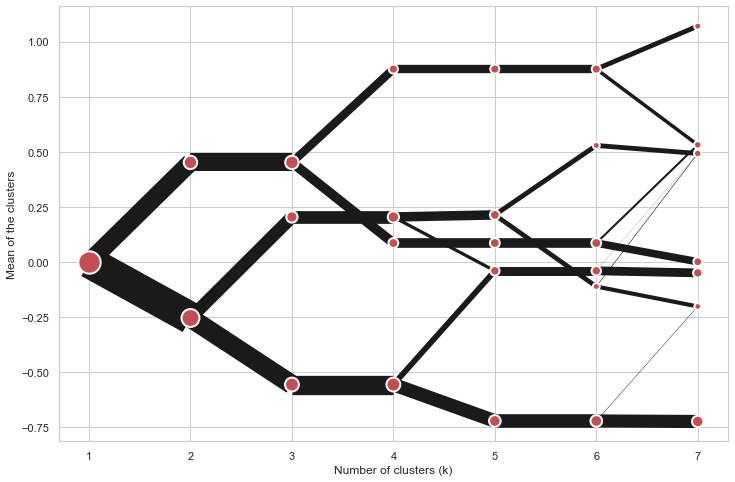## Scikit-learn and RAPIDS cuML backends¶

Clustergram offers two backends for the computation - `scikit-learn` which uses CPU and RAPIDS.AI `cuML`, which uses GPU. Note that both are optional dependencies, but you will need at least one of them to generate clustergram.

Using `scikit-learn` (default):

```cgram = Clustergram(range(1, 8), backend='sklearn')
cgram.fit(data)
cgram.plot()
```

Using `cuML`:

```cgram = Clustergram(range(1, 8), backend='cuML')
cgram.fit(data)
cgram.plot()
```

`data` can be all data types supported by the selected backend (including `cudf.DataFrame` with `cuML` backend).

## Supported methods¶

Clustergram currently supports K-Means, Mini Batch K-Means and Gaussian Mixture Model clustering methods. Note tha GMM and Mini Batch K-Means are supported only for `scikit-learn` backend.

Using K-Means (default):

```cgram = Clustergram(range(1, 8), method='kmeans')
cgram.fit(data)
cgram.plot()
```

Using Mini Batch K-Means, which can provide significant speedup over K-Means:

```cgram = Clustergram(range(1, 8), method='minibatchkmeans', batch_size=100)
cgram.fit(data)
cgram.plot()
```

Using Gaussian Mixture Model:

```cgram = Clustergram(range(1, 8), method='gmm')
cgram.fit(data)
cgram.plot()
```

## Partial plot¶

`Clustergram.plot()` can also plot only a part of the diagram, if you want to focus on a limited range of `k`.

```cgram = Clustergram(range(1, 20))
cgram.fit(data)
cgram.plot(figsize=(12, 8))
```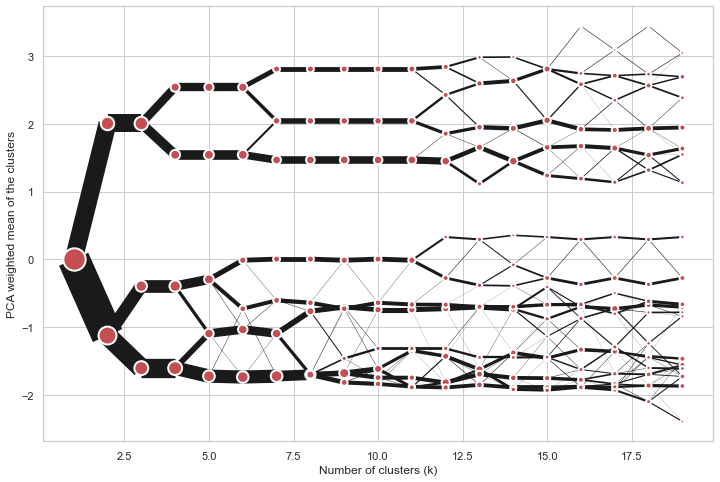```cgram.plot(k_range=range(3, 10), figsize=(12, 8))
```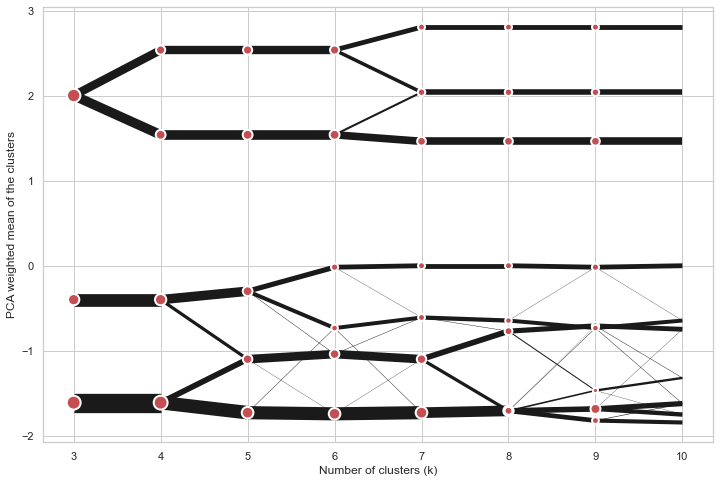Clustergam includes handy wrappers around a selection of clustering performance metrics offered by `scikit-learn`. Data which were originally computed on GPU are converted to numpy on the fly.

### Silhouette score¶

Compute the mean Silhouette Coefficient of all samples. See `scikit-learn` documentation for details.

```>>> cgram.silhouette_score()
2    0.531540
3    0.447219
4    0.400154
5    0.377720
6    0.372128
7    0.331575
Name: silhouette_score, dtype: float64
```

Once computed, resulting Series is available as `cgram.silhouette`. Calling the original method will recompute the score.

### Calinski and Harabasz score¶

Compute the Calinski and Harabasz score, also known as the Variance Ratio Criterion. See `scikit-learn` documentation for details.

```>>> cgram.calinski_harabasz_score()
2    482.191469
3    441.677075
4    400.392131
5    411.175066
6    382.731416
7    352.447569
Name: calinski_harabasz_score, dtype: float64
```

Once computed, resulting Series is available as `cgram.calinski_harabasz`. Calling the original method will recompute the score.

### Davies-Bouldin score¶

Compute the Davies-Bouldin score. See `scikit-learn` documentation for details.

```>>> cgram.davies_bouldin_score()
2    0.714064
3    0.943553
4    0.943320
5    0.973248
6    0.950910
7    1.074937
Name: davies_bouldin_score, dtype: float64
```

Once computed, resulting Series is available as `cgram.davies_bouldin`. Calling the original method will recompute the score.

## Acessing labels¶

`Clustergram` stores resulting labels for each of the tested options, which can be accessed as:

```>>> cgram.labels
1  2  3  4  5  6  7
0    0  0  2  2  3  2  1
1    0  0  2  2  3  2  1
2    0  0  2  2  3  2  1
3    0  0  2  2  3  2  1
4    0  0  2  2  0  0  3
..  .. .. .. .. .. .. ..
337  0  1  1  3  2  5  0
338  0  1  1  3  2  5  0
339  0  1  1  1  1  1  4
340  0  1  1  3  2  5  5
341  0  1  1  1  1  1  5
```

## Saving clustergram¶

You can save both plot and `clustergram.Clustergram` to a disk.

### Saving plot¶

`Clustergram.plot()` returns matplotlib axis object and as such can be saved as any other plot:

```import matplotlib.pyplot as plt

cgram.plot()
plt.savefig('clustergram.svg')
```

### Saving object¶

If you want to save your computed `clustergram.Clustergram` object to a disk, you can use `pickle` library:

```import pickle

with open('clustergram.pickle','wb') as f:
pickle.dump(cgram, f)
```

```with open('clustergram.pickle','rb') as f: